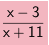### Home > CAAC > Chapter 11 > Lesson 11.1.2 > Problem11-25

11-25.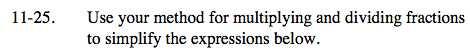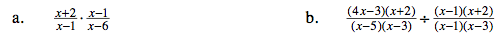Multiply and look for factors that make 1.

Dividing by a fraction is the
same as multiplying by its reciprocal.

Multiply the fractions and look for factors that make one.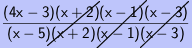$\frac{(4\textit{x}-3)}{(\textit{x}-5)}$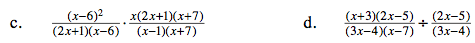See part (b).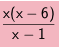See part (b).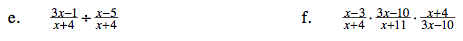See part (b).

See part (b).